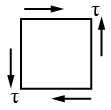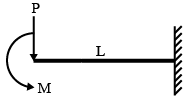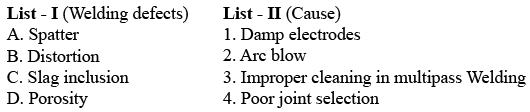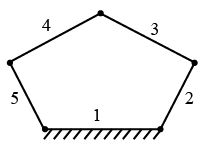# ISRO Scientist or Engineer Mechanical 2011

Instructions

For the following questions answer them individually

Question 51

# For a column of length L is fixed at both ends, corresponding Euler’s critical load isQuestion 52

# Which one of the following figures is the correct sketch of Mohr’s circle of the given state of stressQuestion 53

# With a punch for which the maximum crushing stress is 4 times the maximum shearing stress of the plate, the biggest hole that can be punchedi in the plate would be of diameter equal toQuestion 54

# A simply supported beam with width ‘b’ and. depth ‘d’ carries a central load W and undergoes deflection $$\delta$$ at the centre. If the width and depth are interchanged, the deflection at the centre of the beam would attain the value:Question 55

# The given figure shows a cantilever of span ‘L’ subjected to a concentrated load ‘P’ and a moment ‘M’ at the free end. Deflection at the free end is given byQuestion 56

# In arc welding, penetration is minimum forQuestion 57

# Match list - I (welding effects) with list II (causes) and select the correct answer using the codes given below the lists:Question 58

# Two beams of equal cross sectional area are subjected to equal bending moment. If one beam has square section and the other has circular section then,Question 59

# The number of degree of freedom of a five link plane mechanism with five revolute pairs as shown in the figure isQuestion 60

# To ensure self locking in a screw jack, it is essential that helix angle isOR